Next: Potential Outside a Uniform Up: Gravitational Potential Theory Previous: Axially Symmetric Mass Distributions

Potential Due to a Uniform Sphere

Let us calculate the gravitational potential generated by a sphere of uniform mass density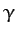and radius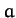. Expressing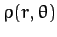in the form (891), it is clear that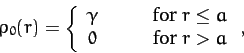(894)

with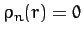for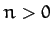. Thus, from (893),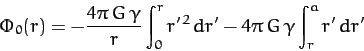(895)

for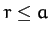, and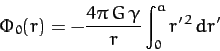(896)

for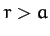, with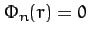for. Hence,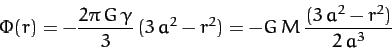(897)

for, and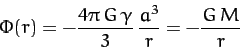(898)

for. Here,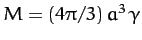is the total mass of the sphere.

According to Equation (898), the gravitational potential outside a uniform sphere of mass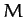is the same as that generated by a point masslocated at the sphere's center. It turns out that this is a general result for any finite spherically symmetric mass distribution. Indeed, from the previous analysis, it is clear that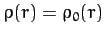and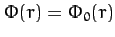for a spherically symmetric mass distribution. Suppose that the mass distribution extends out to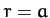. It immediately follows, from Equation (893), that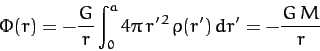(899)

for, whereis the total mass of the distribution. We, thus, conclude that Newton's laws of motion, in their primitive form, apply not only to point masses, but also to extended spherically symmetric masses. In fact, this is something which we have implicitly assumed all along in this book.

According to Equation (897), the gravitational potential inside a uniform sphere is quadratic in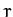. This implies that if a narrow shaft were drilled though the center of the sphere then a test mass,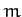, moving in this shaft would experience a gravitational force acting toward the center which scales linearly in. In fact, the force in question is given by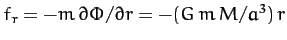. It follows that a test mass dropped into the shaft executes simple harmonic motion about the center of the sphere with period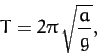(900)

where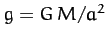is the gravitational acceleration at the sphere's surface.Next: Potential Outside a Uniform Up: Gravitational Potential Theory Previous: Axially Symmetric Mass Distributions
Richard Fitzpatrick 2011-03-31